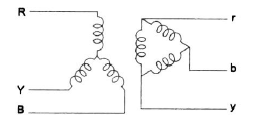Courses

# Test: Power Systems - 3

## 25 Questions MCQ Test Electrical Engineering SSC JE (Technical) | Test: Power Systems - 3

Description
This mock test of Test: Power Systems - 3 for Electrical Engineering (EE) helps you for every Electrical Engineering (EE) entrance exam. This contains 25 Multiple Choice Questions for Electrical Engineering (EE) Test: Power Systems - 3 (mcq) to study with solutions a complete question bank. The solved questions answers in this Test: Power Systems - 3 quiz give you a good mix of easy questions and tough questions. Electrical Engineering (EE) students definitely take this Test: Power Systems - 3 exercise for a better result in the exam. You can find other Test: Power Systems - 3 extra questions, long questions & short questions for Electrical Engineering (EE) on EduRev as well by searching above.
QUESTION: 1

Solution:
QUESTION: 2

Solution:
QUESTION: 3

### Which portion of the transmission system is more prone to faults ?

Solution:
QUESTION: 4

Which portion of the power system is least prone to faults ?

Solution:
QUESTION: 5

The load currents in short-circuit calculation are neglected because
1. short-circuit currents are much larger than load currents.
2. short-circuit currents are greatly out of phase with load currents.
Which of these statement(s) is/are correct ?

Solution:
QUESTION: 6

The symmetrical components are used in the fault analysis because

Solution:
QUESTION: 7

In a star-connected system without neutral grounding, zero-sequence currents are

Solution:
QUESTION: 8

A balanced 3-phase system consists of

Solution:
QUESTION: 9

The positive sequence current of a transmission line is :

Solution:
QUESTION: 10

Zero-sequence fault current is absent when fault is

Solution:
QUESTION: 11

If the positive,-negative-and zero-sequence reactances of an element of a power system are 0.3, 0.3 and 0.8 pu respectively, then the element would be a

Solution:
QUESTION: 12

Negative-sequence reactance of a transformer is

Solution:
QUESTION: 13

The zero-sequence circuit of the three-phase transformer show in the figure isSolution:
QUESTION: 14

A fault occurring at the terminals of an unloaded synchronous generator operating at its rate voltage has resulted in the following values of currents and voltages Ia0 = j2.37 pu, Ia1 = –j3.05 pu, Ia2 = j0.68 pu,Va0 = Va1 = Va2 = 0.237 pu

Solution:
QUESTION: 15

Solution:
QUESTION: 16

The primary function of a fuse is to

Solution:
QUESTION: 17

The material used in liquid fuses is

Solution:
QUESTION: 18

In comparison to rewirable fuses HRC fuses have the advantage(s) of

Solution:
QUESTION: 19

In HRC fuse the time between cut-off and final current zero is called the

Solution:
QUESTION: 20

If a combination of HRC fuse and a circuit breaker is employed, the circuit breaker operates for

Solution:
QUESTION: 21

For a circuit breaker 'break time' is

Solution:
QUESTION: 22

When a fault occurs in a high voltage transmission line, first the

Solution:
QUESTION: 23

The initiation of electric arc at the instant of contact separation is caused by

Solution:
QUESTION: 24

Arcing voltage will be the least in case of

Solution:
QUESTION: 25

The most common type of fault is

Solution:

A single line to ground (LG) fault is one of the most common faults and experiences show that 70-80 percent of the faults that occur in power system are of this type. This forms a short circuit path between the line and ground. These are very less severe faults compared to other faults.